# Specific requirements

In this programming project, complete the following steps:
• Select a data set and load it into Python/R.
• Build a multi-layer deep network to analyze the data of your own choosing.
• Submit a *.html file generated by Jupyter Notebook/R Markdown.

# Data frame information

### Description

Dataset of Social media ads describing users, whether users have purchased a product by clicking on the advertisements shown to them.

### Content

Social media ads of 400 rows and 5 columns.

#### User ID

Character field which catpures the user account number.

#### Gender

Character field which indicates whether the user is female or male.

#### Age

The age of the user.

#### Estimated Salary

The estimated salary of the user.

#### Purchased

A binary field which indicates whether the user made the purchase.

# Clearing R Studio Memory Usage

gc()
##           used (Mb) gc trigger (Mb) max used (Mb)
## Ncells  543094 29.1    1241031 66.3   621331 33.2
## Vcells 1024758  7.9    8388608 64.0  1600889 12.3
rm(list = ls())

# Time Counter Start

start_time <- Sys.time()

# Include the knitr package for integration of R code into Markdown

knitr::opts_chunk$set(echo = TRUE) # All the libraries used in this code library(easypackages) libraries("caret","caretEnsemble","caTools","class","cluster","data.tree","devtools","doSNOW","dplyr","e1071","factoextra","gbm","FNN","FSelector","ggalt","ggforce","ggfortify","ggplot2","gmodels","klaR","lattice","mlbench","modeest","nnet","neuralnet","outliers","parallel","psych","purrr","readr","rpart","rpart.plot","spatialEco","stats","tidyr","randomForest","ROSE","rsample","ROCR","pROC","glmnet","gridExtra","R6") # Import data oldw <- getOption("warn") options(warn = -1) library(readr) input_data <- read_csv("Social_Network_Ads.csv", col_types = cols( Age = col_number(), EstimatedSalary = col_number(), Gender = col_character(), Purchased = col_character(), User ID = col_character() ) ) options(warn = -1)  # Numeric/character field separator num.names <- input_data %>% select_if(is.numeric) %>% colnames() ch.names <- input_data %>% select_if(is.character) %>% colnames() # Descriptive statistics before data processing ### Dimension of data frame dim(input_data) ##  400 5 ### Structure of data frame str(input_data) ## tibble [400 x 5] (S3: spec_tbl_df/tbl_df/tbl/data.frame) ##$ User ID        : chr [1:400] "15624510" "15810944" "15668575" "15603246" ...
##  $Gender : chr [1:400] "Male" "Male" "Female" "Female" ... ##$ Age            : num [1:400] 19 35 26 27 19 27 27 32 25 35 ...
##  $EstimatedSalary: num [1:400] 19000 20000 43000 57000 76000 58000 84000 150000 33000 65000 ... ##$ Purchased      : chr [1:400] "0" "0" "0" "0" ...
##  - attr(*, "spec")=
##   .. cols(
##   ..   User ID = col_character(),
##   ..   Gender = col_character(),
##   ..   Age = col_number(),
##   ..   EstimatedSalary = col_number(),
##   ..   Purchased = col_character()
##   .. )

### Summary statistics of data frame

summary(input_data)
##    User ID             Gender               Age        EstimatedSalary
##  Length:400         Length:400         Min.   :18.00   Min.   : 15000
##  Class :character   Class :character   1st Qu.:29.75   1st Qu.: 43000
##  Mode  :character   Mode  :character   Median :37.00   Median : 70000
##                                        Mean   :37.66   Mean   : 69743
##                                        3rd Qu.:46.00   3rd Qu.: 88000
##                                        Max.   :60.00   Max.   :150000
##   Purchased
##  Length:400
##  Class :character
##  Mode  :character
##
##
## 

### Glimpse of data frame

glimpse(input_data)
## Rows: 400
## Columns: 5
## $User ID <chr> "15624510", "15810944", "15668575", "15603246", "15... ##$ Gender          <chr> "Male", "Male", "Female", "Female", "Male", "Male",...
## $Age <dbl> 19, 35, 26, 27, 19, 27, 27, 32, 25, 35, 26, 26, 20,... ##$ EstimatedSalary <dbl> 19000, 20000, 43000, 57000, 76000, 58000, 84000, 15...
##  37.655
##
##  37
##
##  35
##
##  18
##
##  60
##
##  18 60
##
##  109.8907
##
##  10.48288
##
##  11.8608
##
## $EstimatedSalary ##  31134.6 # Data Processing ## Converting character variables into factors ### Explanation To ensure that R’s data science models work correctly, all categorical dependent variables must be explicitly converted into factors. As for the independent variables, if the variable is both categorical and has more than two levels, then it should be converted into a factor. input_data <- as.data.frame(lapply(input_data, function(x) if(is.character(x)){ x=as.factor(x) } else x)) ## Sorting data set ### Explanation Useful for examinating the data values. By sorting the data, one can tell if there are missing or corrupted data values. input_data <- input_data[order(input_data[,1]),] glimpse(input_data) ## Rows: 400 ## Columns: 5 ##$ User.ID         <fct> 15566689, 15569641, 15570769, 15570932, 15571059, 1...
## $Gender <fct> Female, Female, Female, Male, Female, Female, Male,... ##$ Age             <dbl> 35, 58, 26, 34, 33, 21, 40, 35, 58, 35, 48, 35, 41,...
## $EstimatedSalary <dbl> 57000, 95000, 80000, 115000, 41000, 16000, 71000, 5... ##$ Purchased       <fct> 0, 1, 0, 0, 0, 0, 1, 0, 1, 0, 1, 0, 0, 0, 1, 1, 0, ...
input_data <- input_data[order(-input_data[,1]),]
glimpse(input_data)
## Rows: 400
## Columns: 5
## $User.ID <fct> 15566689, 15569641, 15570769, 15570932, 15571059, 1... ##$ Gender          <fct> Female, Female, Female, Male, Female, Female, Male,...
## $Age <dbl> 35, 58, 26, 34, 33, 21, 40, 35, 58, 35, 48, 35, 41,... ##$ EstimatedSalary <dbl> 57000, 95000, 80000, 115000, 41000, 16000, 71000, 5...
## $Purchased <fct> 0, 1, 0, 0, 0, 0, 1, 0, 1, 0, 1, 0, 0, 0, 1, 1, 0, ... ## Replacing missing values with mean or NA ### Explanation These missing values could cause inaccuracies or errors when calculating data limits, central tendency, dispersion tendency, correlation, multicollinearity, p-values, z-scores, variance inflation factors, etc. input_data <- as.data.frame(lapply(input_data, function(x) if(is.numeric(x) && is.na(x)){ mean(x, na.rm = TRUE) } else { if(is.character(x) && is.na(x)){x = "NA"} else x } )) glimpse(input_data) ## Rows: 400 ## Columns: 5 ##$ User.ID         <fct> 15566689, 15569641, 15570769, 15570932, 15571059, 1...
## $Gender <fct> Female, Female, Female, Male, Female, Female, Male,... ##$ Age             <dbl> 35, 58, 26, 34, 33, 21, 40, 35, 58, 35, 48, 35, 41,...
## $EstimatedSalary <dbl> 57000, 95000, 80000, 115000, 41000, 16000, 71000, 5... ##$ Purchased       <fct> 0, 1, 0, 0, 0, 0, 1, 0, 1, 0, 1, 0, 0, 0, 1, 1, 0, ...

## Scaling numeric variables

### Explanation

These numeric variables are centered and standardized between -1 and 1. In order to correctly calculate the distances between data points, the values of each variable have to be on the same scale. Also, it is easier to fit smaller numbers onto the axes of a graph.

#### Function for standardizing data values

Standardization = (x - mean(x))/std(x)

input_data <- as.data.frame(lapply(input_data, function(x) if(is.numeric(x)){
(x - mean(x)) / sd(x)
} else x))

str(input_data)
## 'data.frame':    400 obs. of  5 variables:
##  $User.ID : Factor w/ 400 levels "15566689","15569641",..: 1 2 3 4 5 6 7 8 9 10 ... ##$ Gender         : Factor w/ 2 levels "Female","Male": 1 1 1 2 1 1 2 2 1 1 ...
##  $Age : num -0.253 1.941 -1.112 -0.349 -0.444 ... ##$ EstimatedSalary: num  -0.374 0.741 0.301 1.327 -0.843 ...
##  $Purchased : Factor w/ 2 levels "0","1": 1 2 1 1 1 1 2 1 2 1 ... glimpse(input_data) ## Rows: 400 ## Columns: 5 ##$ User.ID         <fct> 15566689, 15569641, 15570769, 15570932, 15571059, 1...
## $Gender <fct> Female, Female, Female, Male, Female, Female, Male,... ##$ Age             <dbl> -0.25327018, 1.94078408, -1.11181315, -0.34866384, ...
## $EstimatedSalary <dbl> -0.37371367, 0.74075518, 0.30083327, 1.32731773, -0... ##$ Purchased       <fct> 0, 1, 0, 0, 0, 0, 1, 0, 1, 0, 1, 0, 0, 0, 1, 1, 0, ...

# Descriptive statistics after data processing

### Dimension of data frame

dim(input_data)
##  400   5

### Structure of data frame

str(input_data)
## 'data.frame':    400 obs. of  5 variables:
##  $User.ID : Factor w/ 400 levels "15566689","15569641",..: 1 2 3 4 5 6 7 8 9 10 ... ##$ Gender         : Factor w/ 2 levels "Female","Male": 1 1 1 2 1 1 2 2 1 1 ...
##  $Age : num -0.253 1.941 -1.112 -0.349 -0.444 ... ##$ EstimatedSalary: num  -0.374 0.741 0.301 1.327 -0.843 ...
##  $Purchased : Factor w/ 2 levels "0","1": 1 2 1 1 1 1 2 1 2 1 ... ### Summary statistics of data frame summary(input_data) ## User.ID Gender Age EstimatedSalary Purchased ## 15566689: 1 Female:204 Min. :-1.87496 Min. :-1.605495 0:257 ## 15569641: 1 Male :196 1st Qu.:-0.75409 1st Qu.:-0.784308 1:143 ## 15570769: 1 Median :-0.06248 Median : 0.007552 ## 15570932: 1 Mean : 0.00000 Mean : 0.000000 ## 15571059: 1 3rd Qu.: 0.79606 3rd Qu.: 0.535458 ## 15573452: 1 Max. : 2.13157 Max. : 2.353802 ## (Other) :394 ### Glimpse of data frame glimpse(input_data) ## Rows: 400 ## Columns: 5 ##$ User.ID         <fct> 15566689, 15569641, 15570769, 15570932, 15571059, 1...
## $Gender <fct> Female, Female, Female, Male, Female, Female, Male,... ##$ Age             <dbl> -0.25327018, 1.94078408, -1.11181315, -0.34866384, ...
## $EstimatedSalary <dbl> -0.37371367, 0.74075518, 0.30083327, 1.32731773, -0... ##$ Purchased       <fct> 0, 1, 0, 0, 0, 0, 1, 0, 1, 0, 1, 0, 0, 0, 1, 1, 0, ...

head(input_data)
##    User.ID Gender        Age EstimatedSalary Purchased
## 1 15566689 Female -0.2532702      -0.3737137         0
## 2 15569641 Female  1.9407841       0.7407552         1
## 3 15570769 Female -1.1118131       0.3008333         0
## 4 15570932   Male -0.3486638       1.3273177         0
## 5 15571059 Female -0.4440575      -0.8429637         0
## 6 15573452 Female -1.5887815      -1.5761669         0

### Tail of data frame

tail(input_data)
##      User.ID Gender        Age EstimatedSalary Purchased
## 395 15811613 Female -0.1578765       0.1541926         0
## 396 15813113   Male  0.2236981       1.0926927         1
## 397 15814004   Male -1.0164195      -1.4588544         0
## 398 15814553   Male  1.8453904      -0.2857293         1
## 399 15814816   Male -0.6348448      -0.1097605         0
## 400 15815236 Female  0.7006665       1.7965678         1

### Variable data types

sapply(input_data,mode)
##         User.ID          Gender             Age EstimatedSalary       Purchased
##       "numeric"       "numeric"       "numeric"       "numeric"       "numeric"

### Mean of each variable

lapply(input_data[,num.names],mean)
## $Age ##  -1.050681e-16 ## ##$EstimatedSalary
##  6.539365e-18

### Median of each variable

lapply(input_data[,num.names],median)
## $Age ##  -0.06248285 ## ##$EstimatedSalary
##  0.007551993

### Mode of each variable

lapply(input_data[,num.names],mfv)
## $Age ##  -0.2532702 ## ##$EstimatedSalary
##  0.06620825

### Minimum value of each variable

lapply(input_data[,num.names],min)
## $Age ##  -1.874962 ## ##$EstimatedSalary
##  -1.605495

### Maximum value of each variable

lapply(input_data[,num.names],max)
## $Age ##  2.131571 ## ##$EstimatedSalary
##  2.353802

### Range of each variable

lapply(input_data[,num.names],range)
## $Age ##  -1.874962 2.131571 ## ##$EstimatedSalary
##  -1.605495  2.353802

### Variance of each variable

lapply(input_data[,num.names],var)
## $Age ##  1 ## ##$EstimatedSalary
##  1

### Standard deviation of each variable

lapply(input_data[,num.names],sd)
## $Age ##  1 ## ##$EstimatedSalary
##  1

### Median absolute deviation of each variable

lapply(input_data[,num.names],mad)
## $Age ##  1.131445 ## ##$EstimatedSalary
##  0.9131195

# Plots and Graphs

## Box plots

### Explanation

This box plot reveals the mean value, minimum value, and maximum value of each variable.

### Observation

It appears that both Age and Estimated Salary are pretty much centered at 0. However, if it is broken down by the factor level of the target variable Purchased, each predictor variable appears to have a higher mean for transactions in which the customers did make a purchase.

oldw <- getOption("warn")
options(warn = -1)
boxplot(input_data[,num.names])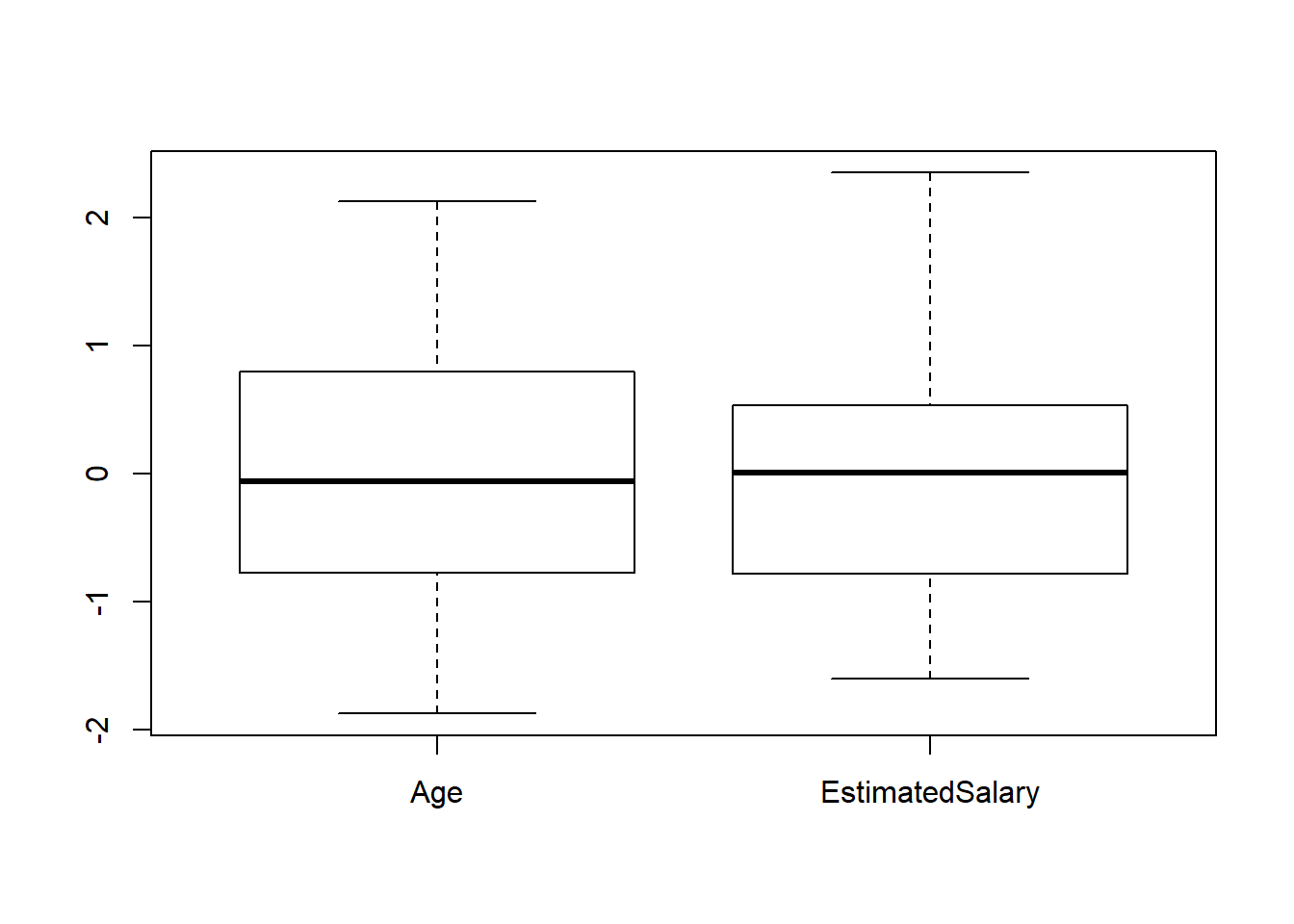options(warn = oldw)
oldw <- getOption("warn")
options(warn = -1)
ggplot(data = input_data, aes(y=Age)) + geom_boxplot(aes(fill=Purchased))+ggtitle("Box Plot of Age")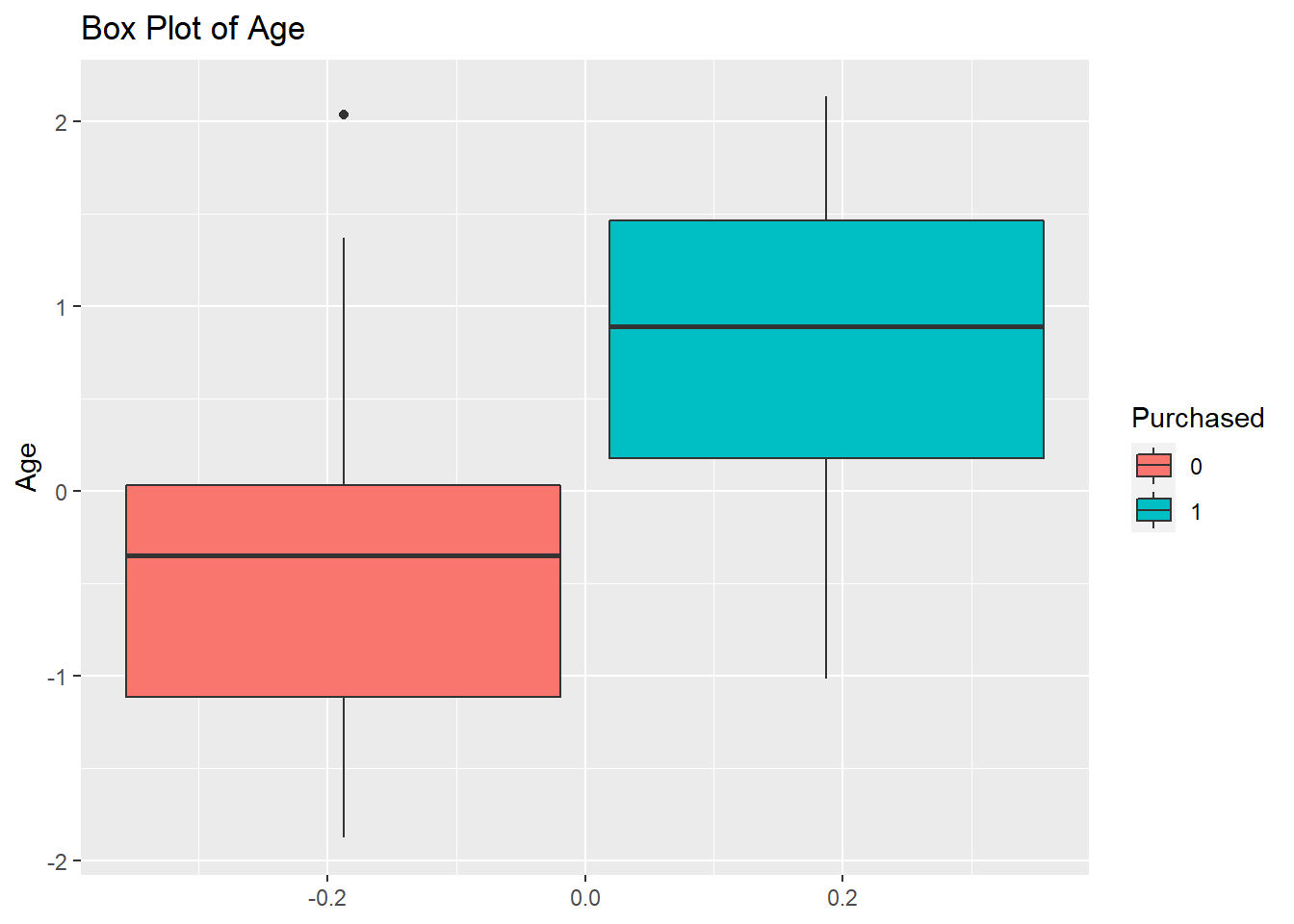ggplot(data = input_data, aes(y=EstimatedSalary)) + geom_boxplot(aes(fill=Purchased))+ggtitle("Box Plot of Estimated Salary")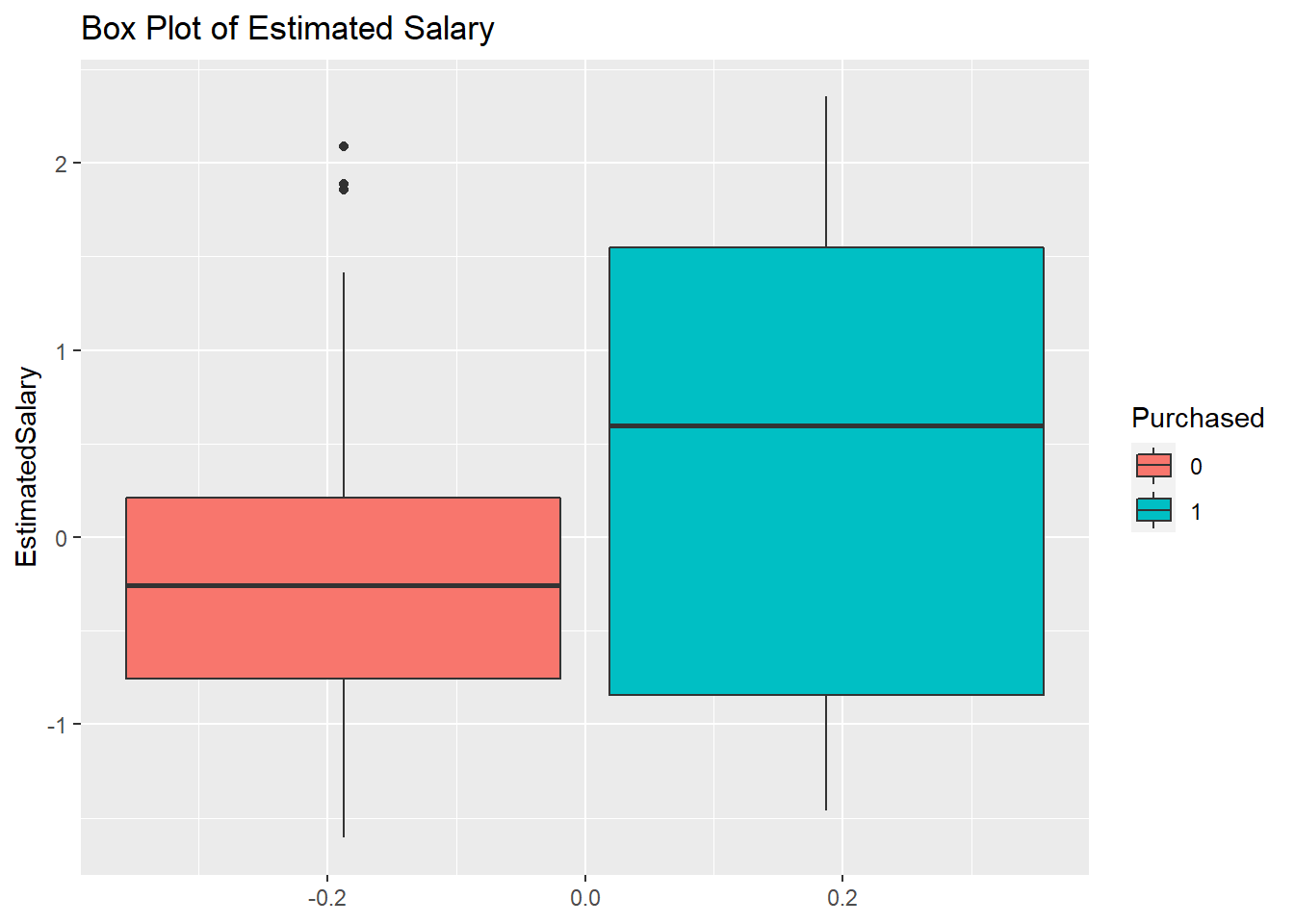options(warn = oldw)

## Histograms

### Explanation

The purpose of these histograms is to see the frequency of each predictor variable under each factor level of the target variable.

### Observation

It appears that the majority of the purchases were made by customers who have low salaries.

oldw <- getOption("warn")
options(warn = -1)
hist(input_data$Age, main = "Histogram of Age", xlab="Age")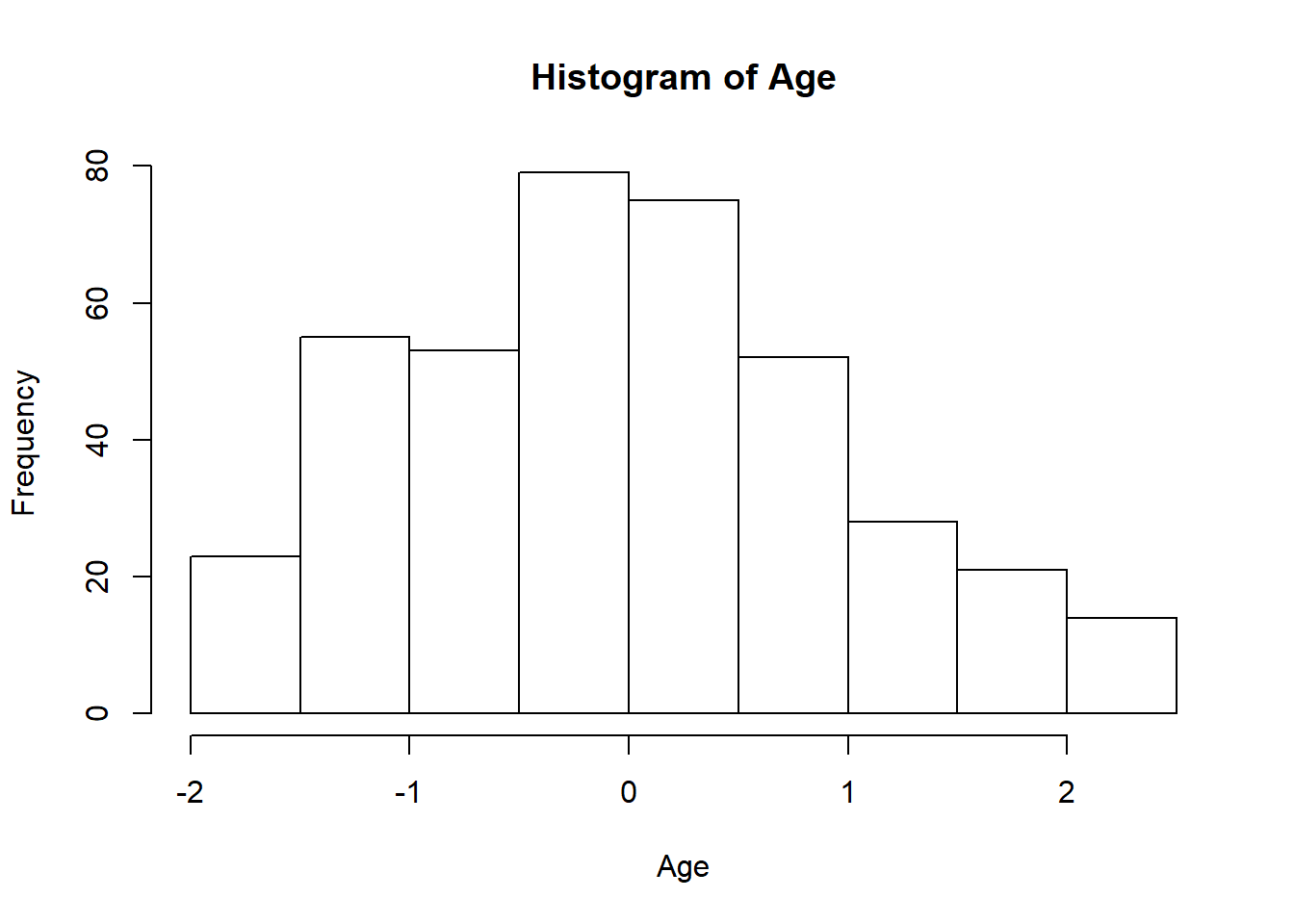hist(input_data$EstimatedSalary, main = "Histogram of Estimated Salary", xlab="Estimated Salary")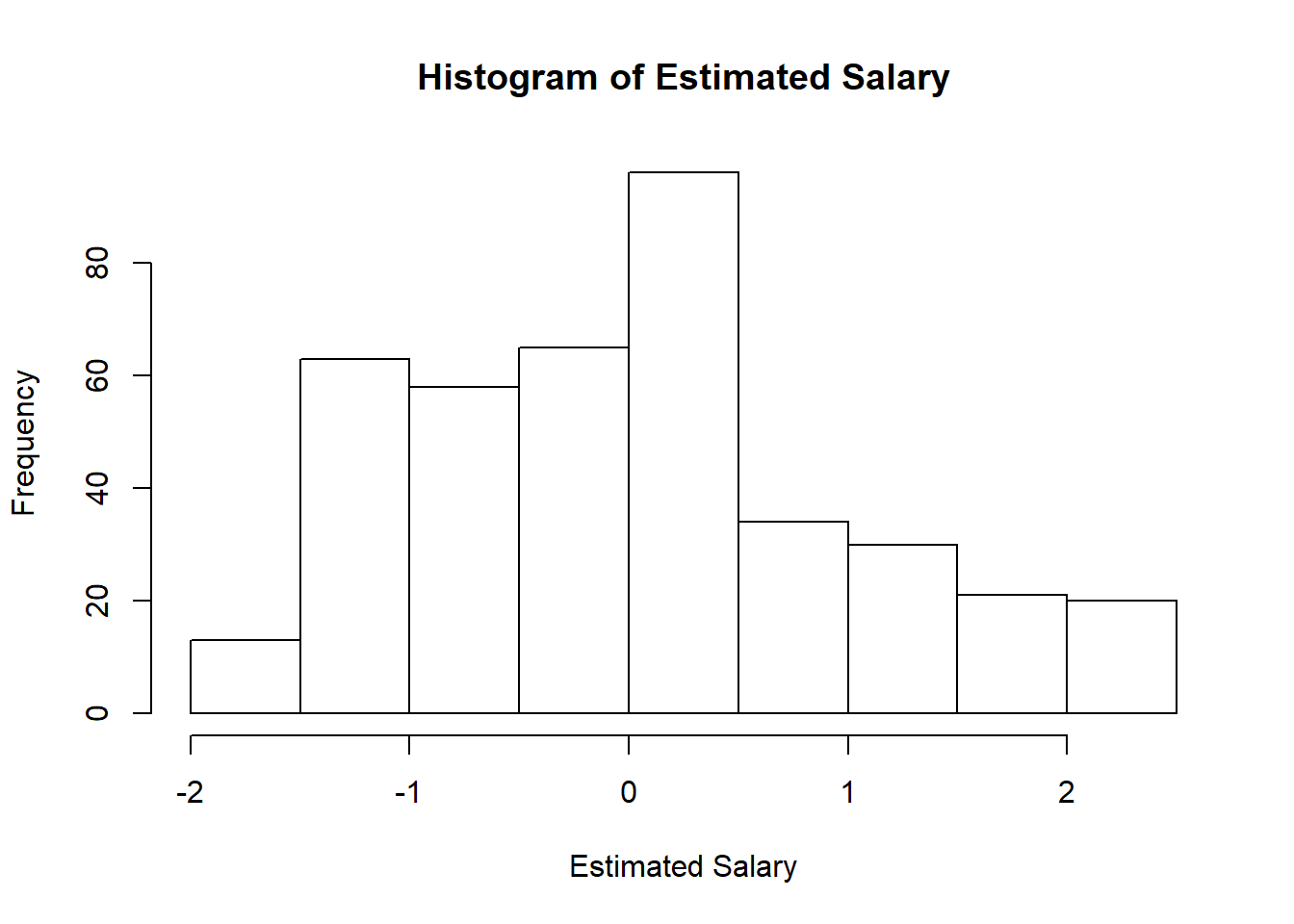options(warn = oldw)
oldw <- getOption("warn")
options(warn = -1)
ggplot(data = input_data, aes(x=Age, fill=Purchased, color=Purchased)) + geom_histogram(alpha=0.6)+ggtitle("Histogram of Age")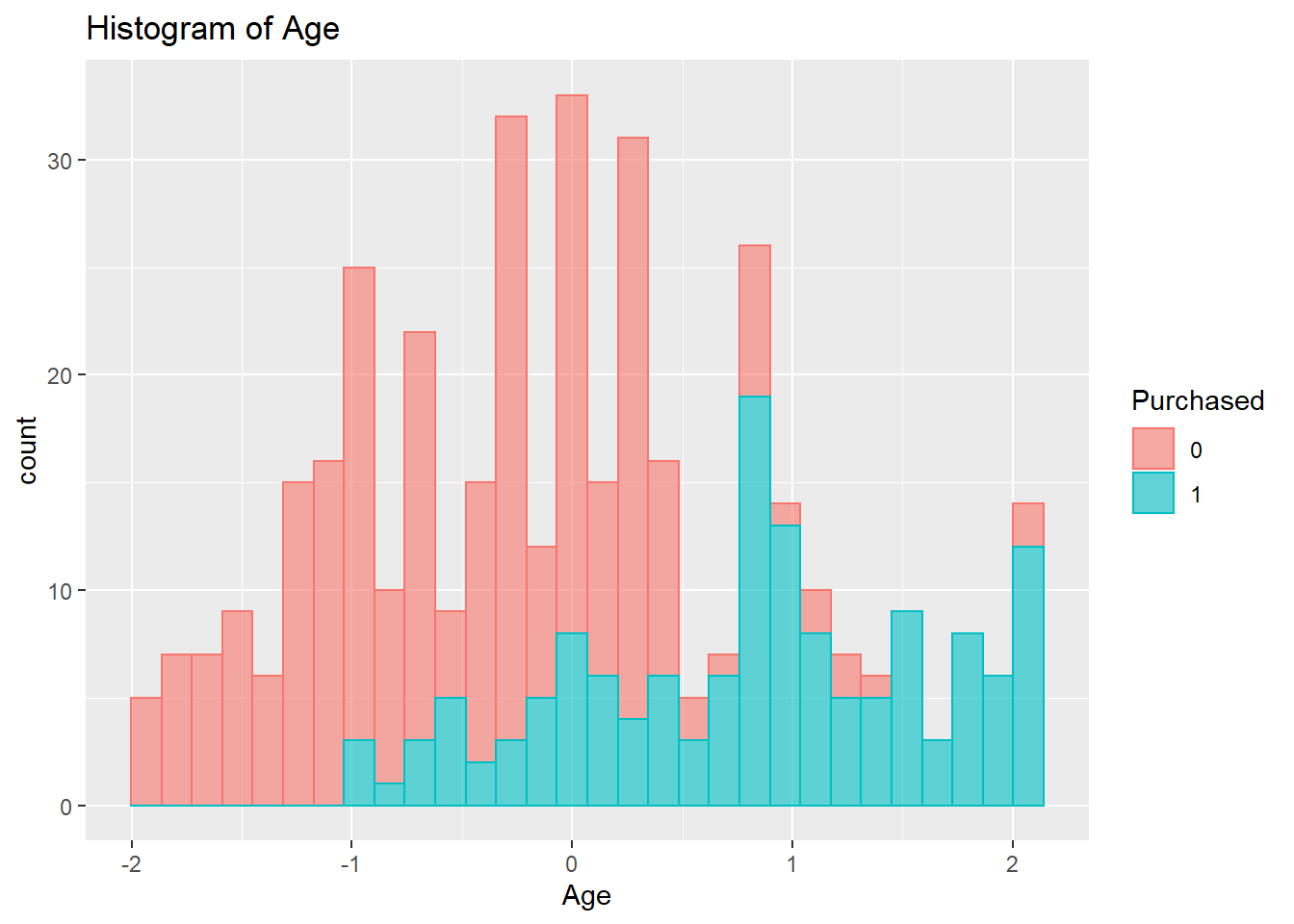ggplot(data = input_data, aes(x=EstimatedSalary, fill=Purchased, color=Purchased)) + geom_histogram(alpha=0.6)+ggtitle("Histogram of Estimated Salary")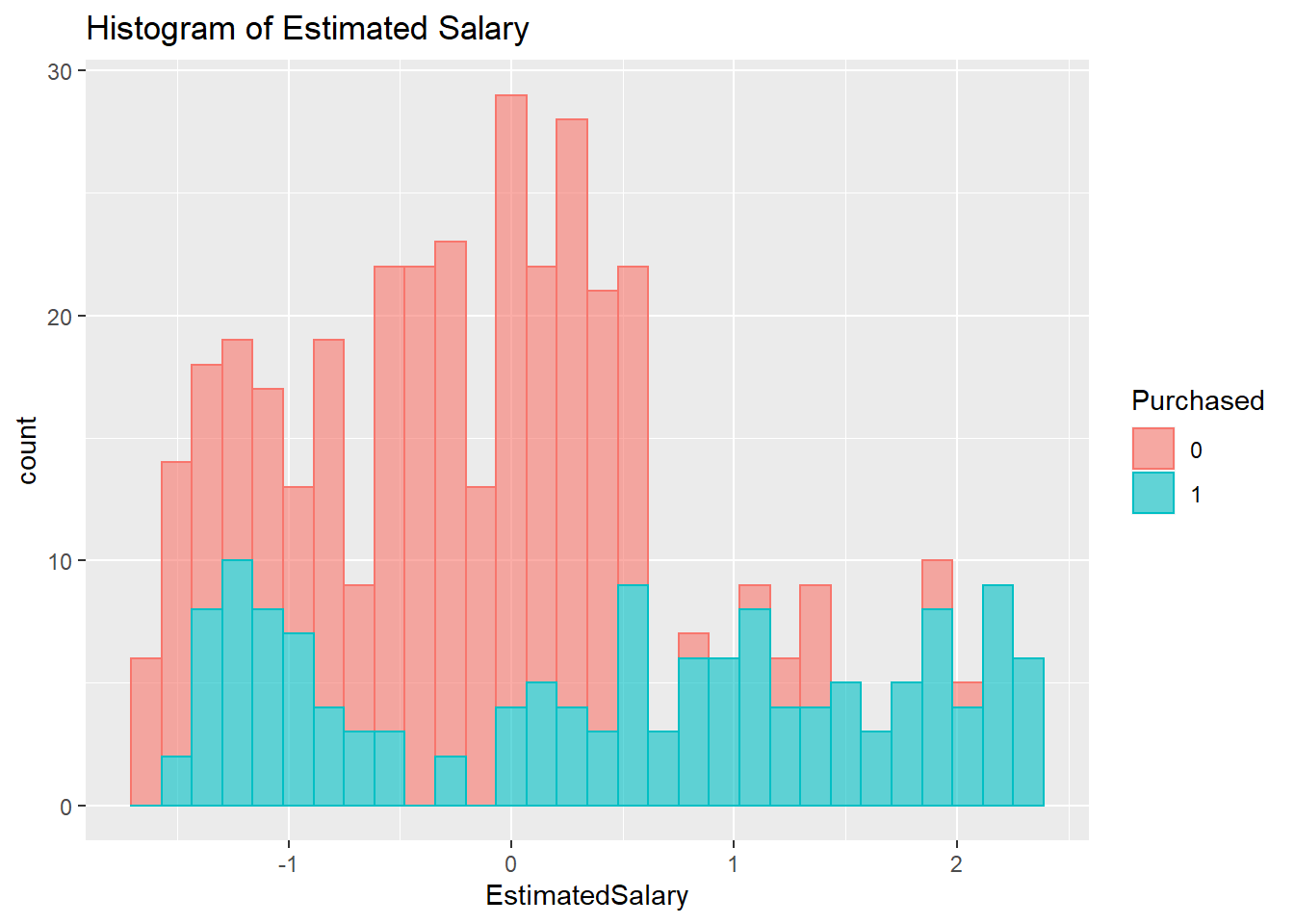options(warn = oldw)

# Grubbs’s Outlier Test

## Explanation

This is a test to check for the existence of outliers associated with each independent variable in the data frame. This test is based on Z-Scores. The function’s null hypothesis is that there are no outliers. If the p-value is smaller than 0.05, then the null hypothesis could be rejected, and the alternative hypothesis that there is at least one outlier could be accepted. The two-tail test is carried out for this data frame.

## Observation

All variables have p-values smaller than 0.05. Given a significant cut-off point of 0.05, all these variables have outliers.

# Detect outliers via z-score
grubbs.test(input_data$Age,two.sided=TRUE,type=11) ## ## Grubbs test for two opposite outliers ## ## data: input_data$Age
## G = 4.0065, U = 0.9798, p-value < 2.2e-16
## alternative hypothesis: -1.87496245115082 and 2.1315714052895 are outliers
grubbs.test(input_data$EstimatedSalary,two.sided=TRUE,type=11) ## ## Grubbs test for two opposite outliers ## ## data: input_data$EstimatedSalary
## G = 3.95930, U = 0.97965, p-value < 2.2e-16
## alternative hypothesis: -1.60549502203623 and 2.35380219630219 are outliers

# Correlation Analysis

## Explanation

The correlation statistics reveal the degree of associations between variables in the data set. Given a range between 0 and 1, a correlation value less than 0.5 in either direction indicates a weak correlation, and a value equal to or greater than 0.5 in either direction indicates a moderate to strong correlation.

## Observation

It appears that none of the variables have correlation coefficient greater than 0.5.

# Correlation Plot

oldw <- getOption("warn")
options(warn = -1)
pairs.panels(input_data[,num.names],gap=0,bg=c("green","red","yellow","blue","pink","purple"),pch= 21, cex=0.5)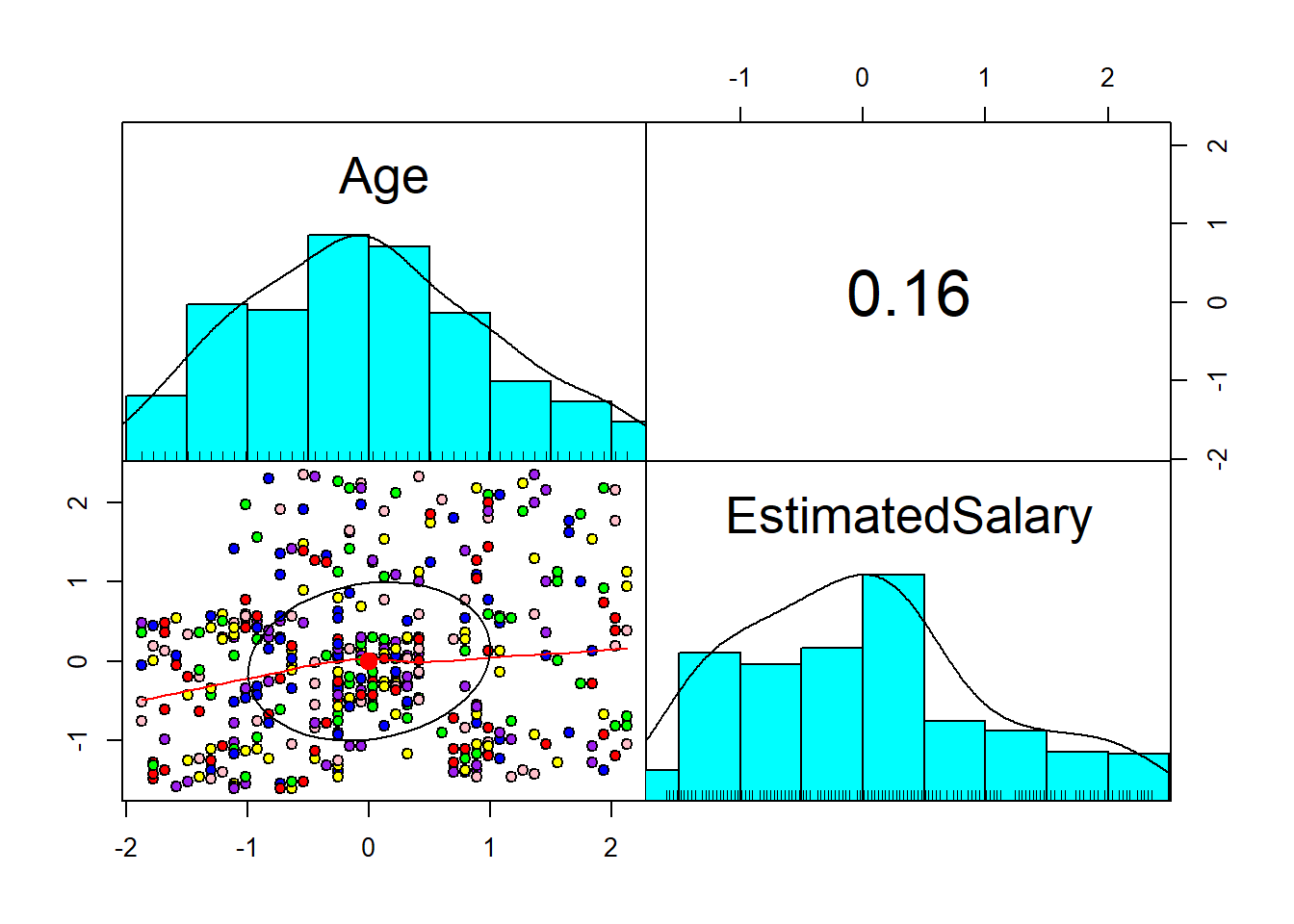options(warn = oldw)

# Correlation Table

oldw <- getOption("warn")
options(warn = -1)
cor(input_data[,num.names])
##                      Age EstimatedSalary
## Age             1.000000        0.155238
## EstimatedSalary 0.155238        1.000000
options(warn = oldw)

# Preparation of Training and Test Data Sets

## Explanation

The purpose of creating separate data sets for training and testing the model is because we want to see how differently the model would perform with data that it has never seen before.

oldw <- getOption("warn")
options(warn = -1)

set.seed(123)
df <- input_data[,c(3,4,5)]
ind <- sample(2, nrow(input_data), replace=T, prob=c(0.6,0.4))
df_sample_train <- df[ind==1,]
df_sample_test <- df[ind==2,]
options(warn = oldw)

# Multi-Layer Perceptrons Neural Network

## Explanation

As the “neural” part of their name suggests, they are brain-inspired systems which are intended to replicate the way that we humans learn. Neural networks consist of input and output layers, as well as (in most cases) a hidden layer consisting of neurons that transform the input into something that the output layer can use. In case of a single-layer perceptrons, there is no hidden layer. They are excellent tools for finding patterns which are far too complex or numerous for a human programmer to extract and teach the machine to recognize.

## Interpretation

Before cross-validation:
After training the model with layers of 5 neurons each, the model predictively ability reached 85.3% accuracy, 89% sensitivity, 83.2% specificity, and a Kappa statistic of 0.69. An accuracy percentage of 85.3% means that the model can predict 85.3% of both the true negatives and true positives. A sensitivity of 89% means that the model can predict 89% of the true positives. A specificity percentage of 83.2% means that the model can predict 83.2% of the true negatives. A Kappa statistic of 0.69 means that the instances classified by the model matched the output labels 69% of the time.
After cross-validation:
After training the model with 10 folds, 10 repeats, tunning length of 20, and 5 layers of neurons, the model predictively ability reached an 85.9% accuracy, 87.27% sensitivity, 85.15% specificity, and a Kappa statistic of 0.7. An accuracy percentage of 85.9% means that the model can predict 85.9% of both the true negatives and true positives. A sensitivity of 87.27% means that the model can predict 87.27% of the true positives. A specificity percentage of 85.15% means that the model can predict 85.15% of the true negatives. A Kappa statistic of 0.7 means that the instances classified by the model matched the output labels 70% of the time.
It appears that the only good thing that cross-validation does for a neural network model is to improve its ability to capture the true negatives. In other words, the only big impact that cross-validation has is to raise the specificity percentage.

## Modeling without cross-validation

oldw <- getOption("warn")
options(warn = -1)

# Making sure the target variable is in numeric format
df_sample_train$Purchased = as.integer(as.character(df_sample_train$Purchased))
df_sample_test$Purchased = as.integer(as.character(df_sample_test$Purchased))

# neuralnet cannot accept y~. as formula
nn_train <- neuralnet(formula=Purchased~Age+EstimatedSalary, data=df_sample_train, hidden=5, act.fct = "logistic", linear.output=FALSE) #linear.output=FALSE is neccessary for classifcation NN

# Neural Network Result
head(as.data.frame(nn_train$result.matrix), n=5) ## V1 ## error 4.084975e+00 ## reached.threshold 9.333901e-03 ## steps 1.121000e+03 ## Intercept.to.1layhid1 1.180124e+01 ## Age.to.1layhid1 9.992873e+00 # Neural Network Plot plot(nn_train, rep="best") #rep="best" must be included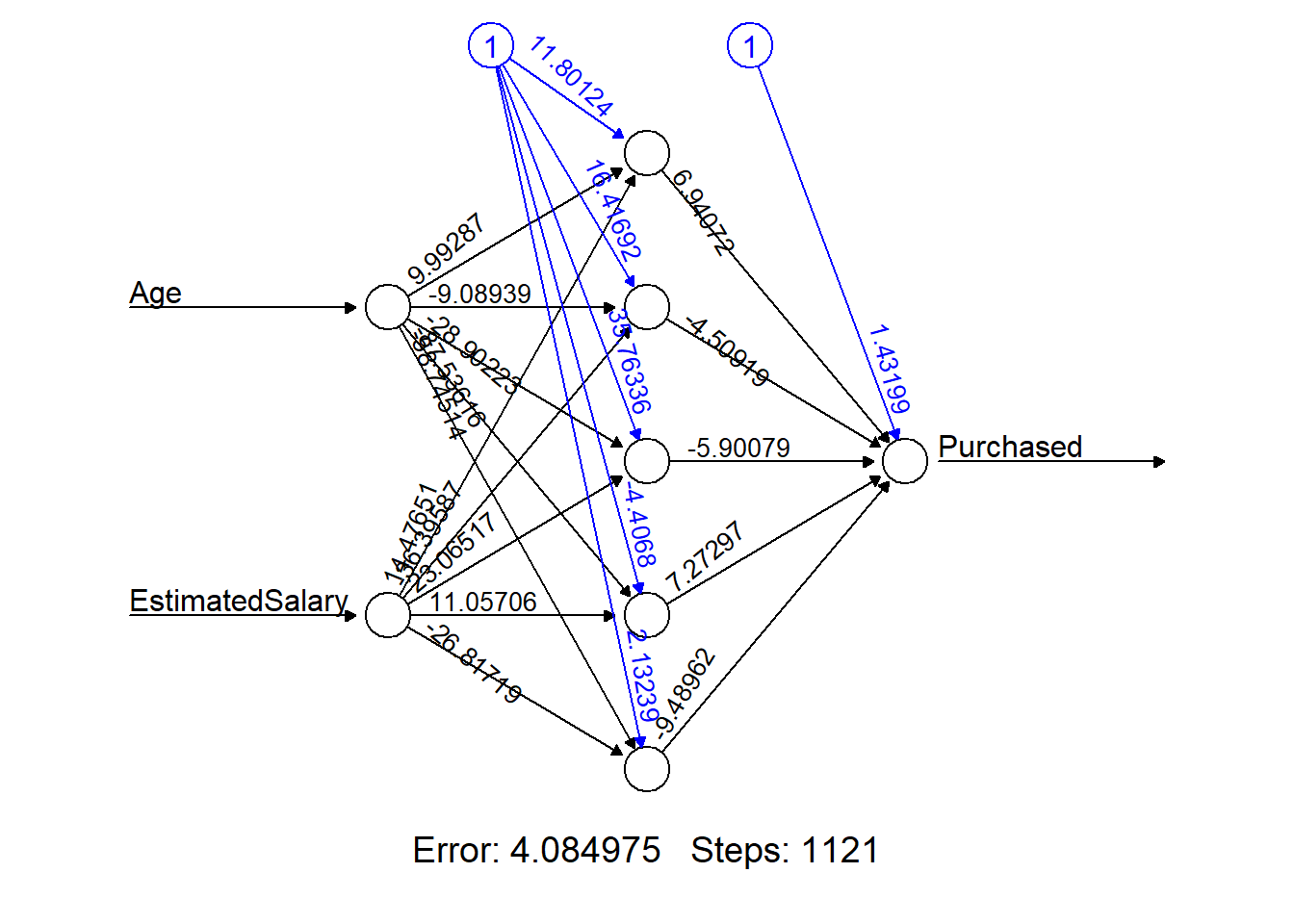# Test the neural network on some test data nn_pred <- compute(nn_train, df_sample_test) options(warn = oldw) ## Confusion Matrix before cross-validation ### Explanation Comparison between the predicted values and actual observed values. Calculate the average accuracy statistics. ### Observation After training the model with 100 repeats and 5 layers of neurons, the model predictively ability reached 85.3% accuracy, 89% sensitivity, 83.2% specificity, and a Kappa statistic of 0.69. oldw <- getOption("warn") options(warn = -1) # nn_pred$net.result
pred_simo <- ifelse(nn_pred$net.result>0.5, 1, 0) # head(pred_simo, n=5) table(pred_simo) ## pred_simo ## 0 1 ## 90 66 table(df_sample_test$Purchased)
##
##   0   1
## 101  55
# table(Predicted=pred_simo,Actual=df_sample_test$Purchased) confusionMatrix( table(Predicted=pred_simo,Actual=df_sample_test$Purchased), positive='1' )
## Confusion Matrix and Statistics
##
##          Actual
## Predicted  0  1
##         0 84  6
##         1 17 49
##
##                Accuracy : 0.8526
##                  95% CI : (0.787, 0.9042)
##     No Information Rate : 0.6474
##     P-Value [Acc > NIR] : 8.4e-09
##
##                   Kappa : 0.6911
##
##  Mcnemar's Test P-Value : 0.03706
##
##             Sensitivity : 0.8909
##             Specificity : 0.8317
##          Pos Pred Value : 0.7424
##          Neg Pred Value : 0.9333
##              Prevalence : 0.3526
##          Detection Rate : 0.3141
##    Detection Prevalence : 0.4231
##       Balanced Accuracy : 0.8613
##
##        'Positive' Class : 1
## 
options(warn = oldw)

## Modeling with cross-validation

### Explanation

The purpose of cross-validation is to prevent overfitting. Overfitting happens when the resulting model performs badly on test data. Meaning, if the data points in the test data set are slightly different from the training data set, the model will not be able to make predictions with acceptable accuracy.

### Observation

After training the model with 10 folds, 10 repeats, tunning length of 20, and 5 layers of neurons, the model predictively ability reached an 85.9% accuracy, 87.27% sensitivity, 85.15% specificity, and a Kappa statistic of 0.7.

oldw <- getOption("warn")
options(warn = -1)

# Set up caret's trainControl object
trainCtrl <- trainControl(method = "repeatedcv", number=10, repeats=10, savePredictions=T, verboseIter=T)
# summary(trainCtrl)

# Set up doSNOW package for multi-core training. This will speed up the training process.
numCores <- detectCores()
c1 <- makeCluster(numCores,type="SOCK")
registerDoSNOW(c1)

# Set seed for reproducibility
set.seed(123)

# Set the number of neurons per hidden layer
tune.grid.neuralnet <- expand.grid(
layer1 = 5,
layer2 = 5,
layer3 = 5
)

train_obj <- train(Purchased~Age+EstimatedSalary, method="neuralnet", data=df_sample_train,  act.fct = "logistic", linear.output=FALSE, tuneGrid = tune.grid.neuralnet, tuneLength=20, metric = "AUC", trControl=trainCtrl)
## Aggregating results
## Fitting final model on full training set
# Shutdown cluster
stopCluster(c1)
plot(varImp(train_obj, scale=T))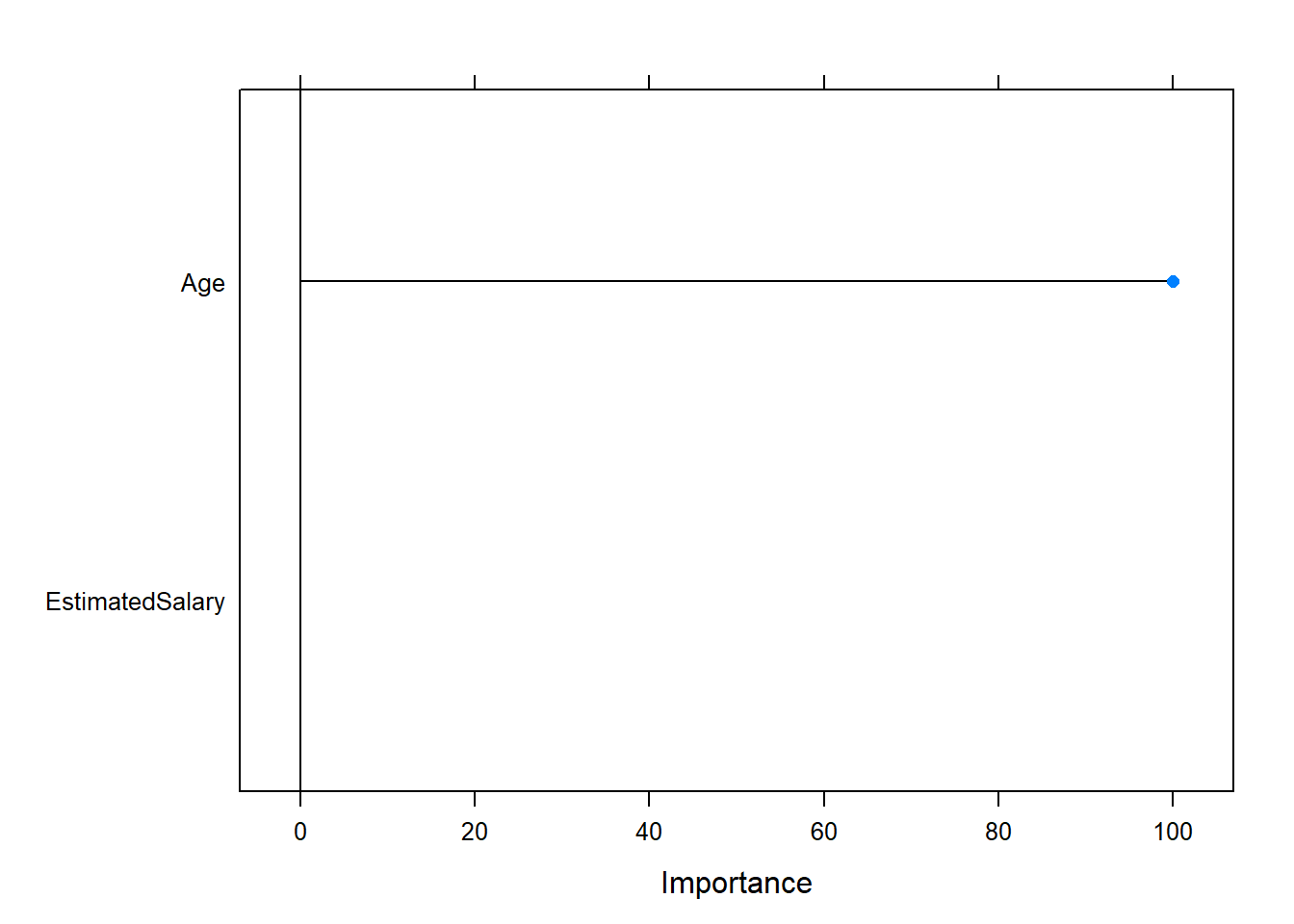pred_roc <- ifelse((train_obj$pred)$pred>0.5, 1, 0)
table(pred_roc)
## pred_roc
##    0    1
##  276 2115
table((train_obj$pred)$obs)
##
##    0    1
## 1560  880
table(Predicted=pred_roc,Actual=(train_obj$pred)$obs) 
##          Actual
## Predicted    0    1
##         0  238   38
##         1 1285  830
confusionMatrix(table(Predicted=pred_roc,Actual=(train_obj$pred)$obs), positive='1')
## Confusion Matrix and Statistics
##
##          Actual
## Predicted    0    1
##         0  238   38
##         1 1285  830
##
##                Accuracy : 0.4467
##                  95% CI : (0.4266, 0.4669)
##     No Information Rate : 0.637
##     P-Value [Acc > NIR] : 1
##
##                   Kappa : 0.0859
##
##  Mcnemar's Test P-Value : <2e-16
##
##             Sensitivity : 0.9562
##             Specificity : 0.1563
##          Pos Pred Value : 0.3924
##          Neg Pred Value : 0.8623
##              Prevalence : 0.3630
##          Detection Rate : 0.3471
##    Detection Prevalence : 0.8846
##       Balanced Accuracy : 0.5562
##
##        'Positive' Class : 1
## 
options(warn = oldw)

## Using the final model to make prediction

oldw <- getOption("warn")
options(warn = -1)
#Test the neural network on some test data
nn_pred <- compute(train_obj$finalModel, df_sample_test) pred_roc <- ifelse(nn_pred$net.result>0.5, 1, 0)
table(pred_roc)
## pred_roc
##  0  1
## 93 63
table(df_sample_test$Purchased) ## ## 0 1 ## 101 55 table(Predicted=pred_roc,Actual=df_sample_test$Purchased)
##          Actual
## Predicted  0  1
##         0 86  7
##         1 15 48
confusionMatrix(table(Predicted=pred_roc,Actual=df_sample_test\$Purchased), positive="1")
## Confusion Matrix and Statistics
##
##          Actual
## Predicted  0  1
##         0 86  7
##         1 15 48
##
##                Accuracy : 0.859
##                  95% CI : (0.7943, 0.9095)
##     No Information Rate : 0.6474
##     P-Value [Acc > NIR] : 2.59e-09
##
##                   Kappa : 0.701
##
##  Mcnemar's Test P-Value : 0.1356
##
##             Sensitivity : 0.8727
##             Specificity : 0.8515
##          Pos Pred Value : 0.7619
##          Neg Pred Value : 0.9247
##              Prevalence : 0.3526
##          Detection Rate : 0.3077
##    Detection Prevalence : 0.4038
##       Balanced Accuracy : 0.8621
##
##        'Positive' Class : 1
## 
options(warn = oldw)

# Process Runtime

end_time <- Sys.time()
end_time - start_time
## Time difference of 3.096243 mins